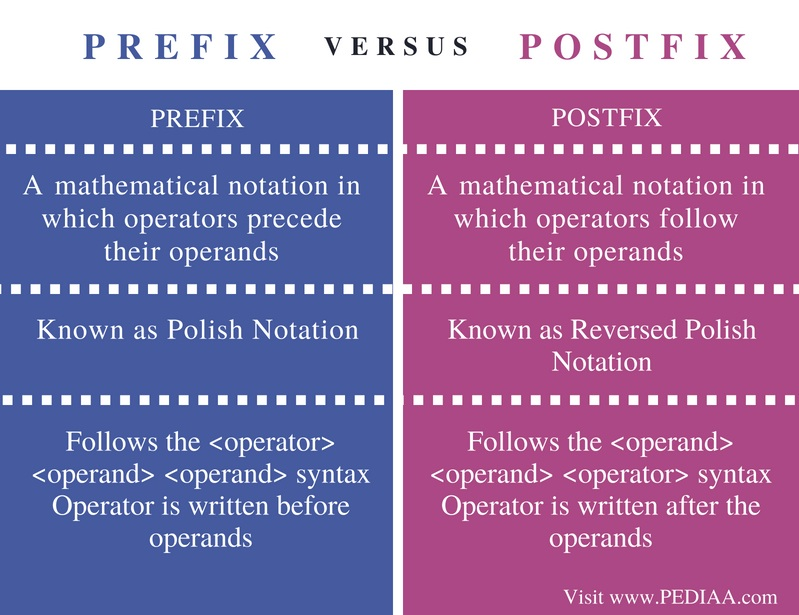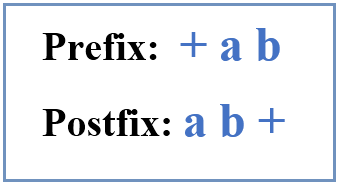# Difference Between Prefix and Postfix

The main difference between prefix and postfix is that the prefix is a notation that writes the operator before operands while the postfix is a notation that writes the operator after the operands.

Notation is the way of writing arithmetic expressions. There are various notations to write an arithmetic expression. They do not change the output of the expression. These notations include infix notation, prefix notation, and postfix notation.

### Key Areas Covered

1. What is Infix
– Definition, Examples
2. What is Prefix
– Definition, Examples
3. What is Postfix
– Definition, Examples
4. Difference Between Prefix and Postfix
– Comparison of Key Differences

### Key Terms

Infix, Prefix, Postfix## What is Infix

Some examples of arithmetic expressions are 4+5, a+b, etc.  They have a common structure as follows.

<operand> <operator>  <operand>

The operator refers to the operation that should be performed while the operand refers to the value the operation is performed on. For example, in a +b expression, ‘a’ and ‘b’ are operands and ‘+’ is the operator.

In these expressions, the operators are used in between the operands. This type of notation is easier for humans to read and understand.  But in computing, the infix notation is not useful as it requires more time and space to process the expressions. Prefix and Postfix notations are the solutions for this issue.

## What is Prefix

In prefix notation, the operator is written before the operands. Prefix notation is also called polish notation.

Some examples of prefix notation are as follows.

a+b is written as + a b

p – q is written as – p q

Refer the below expression.

a + b*c

First, the multiplication will occur as follows. The operands for multiplication are b and c.

a + * b c

Then the addition will occur. The operands for addition are a and * b c. The final prefix notation is as follows.

+ a * b c

## What is Postfix

In postfix notation, the operator is written after the operands. It is also called Reverse Polish Notation.  Some examples for postfix notation are as follows.

a + b is written as a b +

p – q is written as p q –

Refer below expression.

a + b * c

First, the multiplication will occur as follows. The operands for multiplication are b and c.

a +  b c *

Then the addition will occur. The operands for addition are a and b c *. The final prefix notation is as follows.

a b c * +Figure 1: Prefix and Postfix

Overall, humans find it difficult to understand prefix and postfix, but they are easier for the computer to process.

## Difference Between Prefix and Postfix

### Definition

Prefix is a mathematical notation in which operators precede their operands. Postfix is a mathematical notation in which operators follow their operands.

### Synonyms

Also, prefix is known as Polish Notation, and postfix is known as Reversed Polish Notation.

### Syntax

The prefix notation follows the <operator> <operand> <operand> syntax.  In other words, the operator is written before operands. The postfix notation follows the <operand> <operand> <operator> syntax. In other words, the operator is written after the operands.

### Conclusion

Prefix and Postfix are two notations used in computing. The difference between prefix and postfix is that the prefix is a notation that writes the operator before operands while the postfix is a notation that writes the operator after the operands.

##### Reference:

1. “Data Structures and Algorithms Parsing Expressions.” Www.tutorialspoint.com, Tutorials Point, 21 July 2018, Available here.### About the Author: Lithmee

Lithmee holds a Bachelor of Science degree in Computer Systems Engineering and is reading for her Master’s degree in Computer Science. She is passionate about sharing her knowldge in the areas of programming, data science, and computer systems.